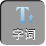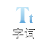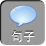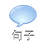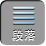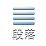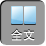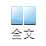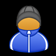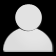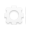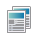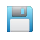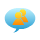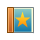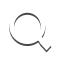-AA+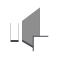（１）已知其余两个角分别是多少，就可以求出这个角；

（２）已知一个角，并且已知所求角和另一个角的关系就可以求出这个角．

∵∠ A＋∠ B＋∠ C180°，

60°＋∠ C10°＋∠ C180°

∴∠ C55°．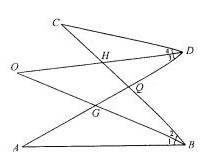C+∠ 4=∠ O+∠ 2

A+∠ 1=∠ O+∠ 3

①+②∠ A+∠ C+∠ 1+∠ 4= 2O+∠ 2+∠ 3

∵∠ 1=∠ 23=∠ 4∴∠ A+∠ C= 2O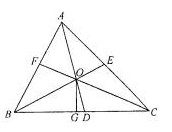∴∠ AOB= 180°－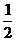(∠ ABC+∠ BAC)= 90°+ACB,

BOD= 180° -AOB= 90°－ACB= 90° -OCD

OGBCG∴∠ GOC= 90° -OCD∴∠ BOD= COG

∴∠ B= 180° -11× 11°= 59°

∴∠ A= 40°∠ B= 60°∠ C= 80°

α= 140°β= 120°γ= 100°

∴α：β：γ= 765

∴α∶β∶γ= 765

A60°＜α≤ 90°

B0°＜α≤ 45°

C0°＜α≤ 30°

D0°＜α≤ 60°

3α≤α+β+γ= 180°

∴α≤ 60°选 D

A．∠ A+∠ D= 90°

B．｜∠ A -D｜= 90°

CA+∠ D= 90°

D．｜A -D｜= 90°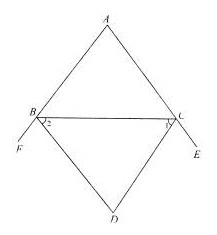1=BCE=(∠ A+∠ ABC)

2=CBF=(∠ A+∠ ACB)

∴∠ D= 180°－(∠ A+∠ ABC+∠ ACB+∠ A)

= 180° -( 180°+∠ A= 90°－A

∴∠ D+A= 90°

C

∴∠ B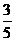A．

3C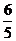AC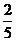A＜∠ A

∴∠ A为最大内角．

180°=∠ A+∠ B+∠ C＜∠ A+A+A= 2A

∴∠ A90°

∴△ ABC为钝角三角形．